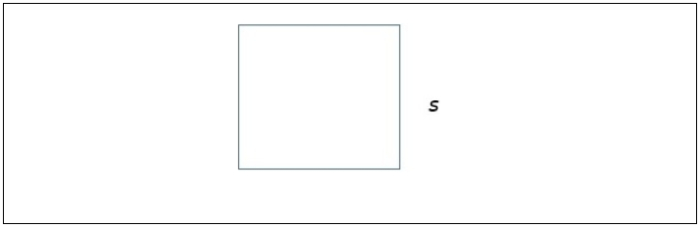# Java Program to Find Area of Square

In this article, we will understand how to find the area of a square. The area of a square is calculated using the following formula −

side*side
i.e.
s2

Below is a demonstration of the same −

If the side of a square is s, the area of the square is given by s2Input

Suppose our input is −

Length of the side : 4


Output

The desired output would be −

Area of the square : 16

## Algorithm

Step 1 - START
Step 2 - Declare 2 integer values namely my_side and my_area.
Step 3 - Read the required values from the user/ define the values.
Step 4 – Calculate the area of the square using the formula side*side and store the result
Step 5- Display the result
Step 6- Stop

## Example 1

Here, the input is being entered by the user based on a prompt. You can try this example live in ourcoding ground tool.

import java.util.Scanner;
public class AreaOfSquare {
public static void main(String args[]){
int my_side, my_area;
System.out.println("Required packages have been imported");
Scanner my_scanner = new Scanner(System.in);
System.out.println("A reader object has been defined ");
System.out.print("Enter the length of a side : ");
my_side = my_scanner.nextInt();
my_area = my_side* my_side;
System.out.println("The my_area of the square is :"+my_area);
}
}

## Output

Required packages have been imported
A reader object has been defined
Enter the length of a side : 4
The area of the square is :16

## Example 2

Here, the integer has been previously defined, and its value is accessed and displayed on the console.

public class AreaOfSquare {
public static void main(String args[]){
int my_side, my_area;
my_side = 4;
System.out.println("The length of the side of the square is defined as : " +my_side);
my_area = my_side* my_side;
System.out.println("The my_area of the square is :"+my_area);
}
}

## Output

The length of the side of the square is defined as : 4
The area of the square is :16